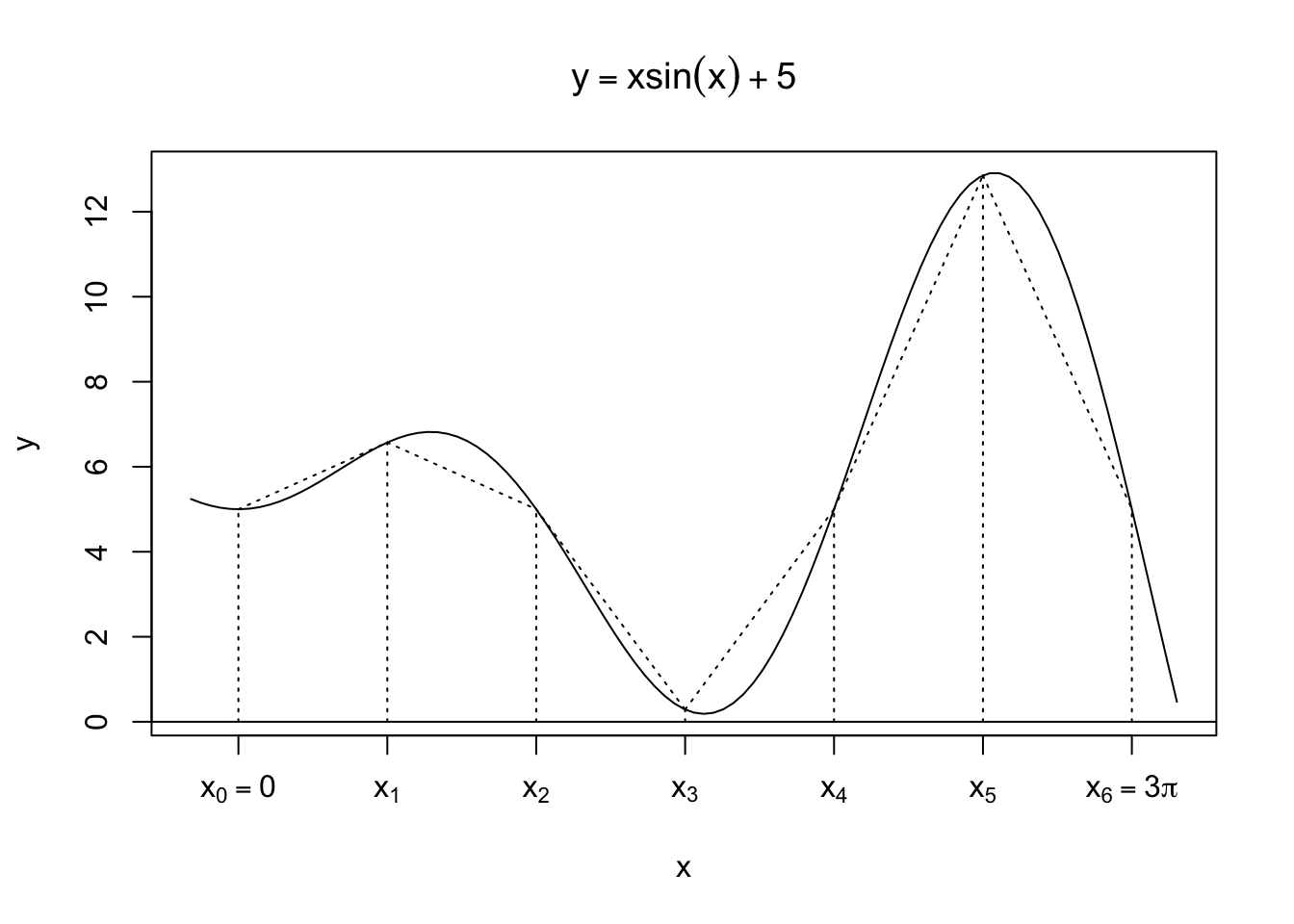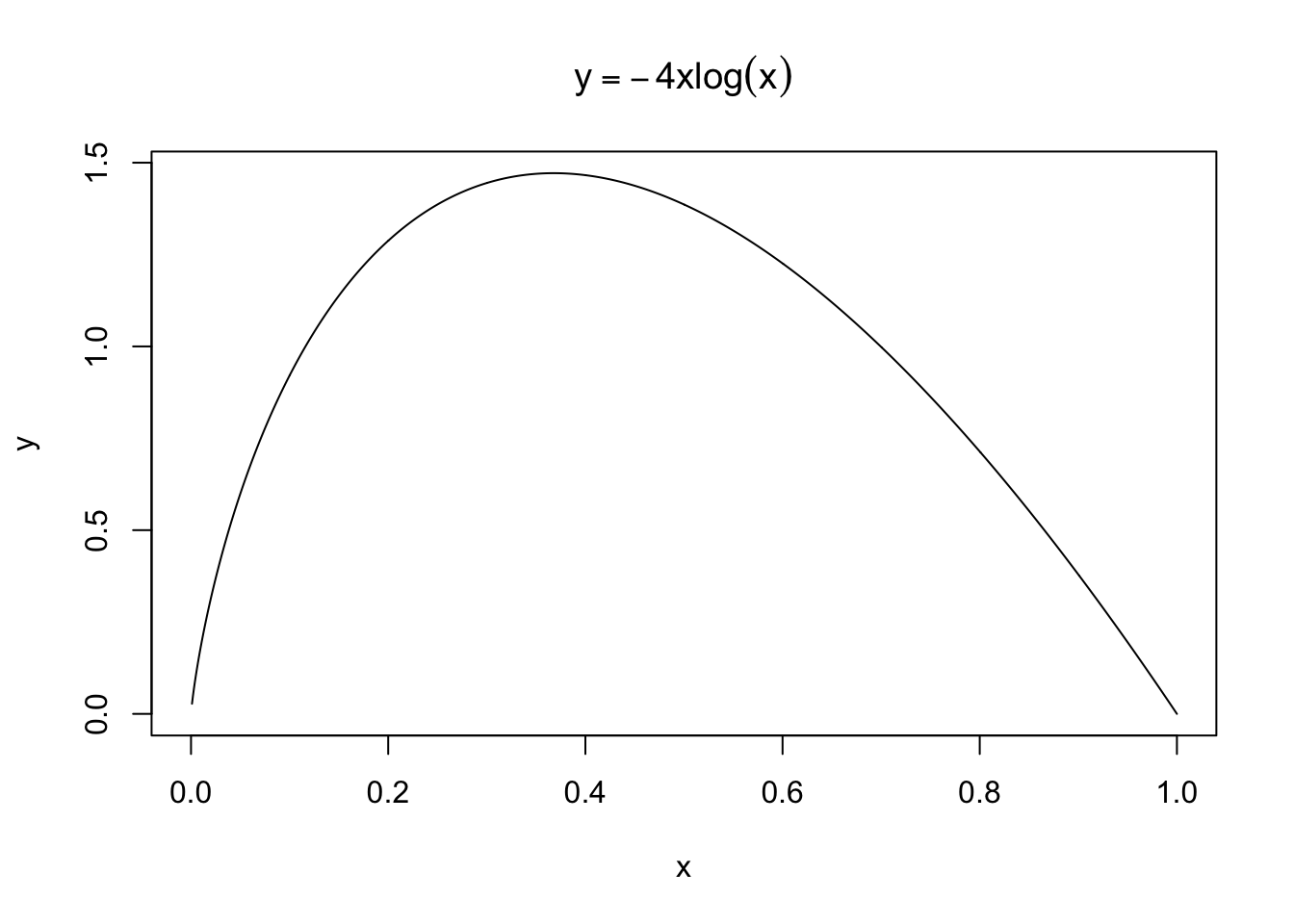These notes are based on Chapter 11 of Jones, O., Maillardet, R., & Robinson, A. (2009). Introduction to scientific programming and simulation using R. CRC Press.

# Introduction

A definite integral $$\int_a^b f(x)dx$$ is easy to compute if we can find an antiderivative $$F$$ such that $$F'(x) = f(x)$$ because $$\int_a^b f(x)dx = F(b)-F(a)$$.

Unfortunately, for many functions, it is impossible to write down an antiderivative in a “closed form” so that we can compute definite integrals without numerical methods.

Numerical integration could provide good approximation of definite integrals. For example, the cumulative distribution function (CDF) of the standard normal distribution $\Phi(z) = \int_{-\infty}^z\frac{1}{\sqrt{2\pi}}e^{-x^2/2}dx$ is commonly computed via numerical integration. R has pnorm for $$\Phi$$ and, for example,

pnorm(-1)
##  0.1586553

is an approximation of $$\Phi(-1)$$.

We cover three numerical integration techniques:

• Trapezoidal rule,
• Simpson’s rule,

These are deterministic methods for numerical integration. In contrast, Monte Carlo integration is a class of stochastic methods.

A test problem is to integrate $$f(x) = x\sin(x)+5$$ over $$[0,3\pi]$$, which can be analytically done using integration by parts. \begin{align} \int_0^{3\pi} x\sin(x)+5 dx &= \int_0^{3\pi} x\sin(x) dx + \int_0^{3\pi} 5 dx\\ &= \left( -x\cos(x)\Bigr\rvert_0^{3\pi} + \int_0^{3\pi}\cos(x)dx \right) + 15\pi\\ &= 18\pi\\ &\approx 56.5486677646 \end{align}

test1 <- function(x) return(x*sin(x)+5)
integrate(test1, 0, 3*pi) # R built-in function
## 56.54867 with absolute error < 8e-12

# Trapezoidal rule

Given a finite set of subintervals, the trapezoidal rule approximates the area under $$y=f(x)$$ by the finite sum of trapezoid areas, which are easy to calculate.

The following is an example with $$n=6$$ intervals.

curve(x*sin(x)+5, xaxt="n", from=-.5, to=9.9, ylab="y")
abline(h=0)
title(expression(y==x*sin(x)+5))
x <- seq(0, 3*pi, .5*pi)
# How can we generate these expressions using a loop?
letters <- c(expression(x==0), expression(x), expression(x),
expression(x), expression(x), expression(x),
expression(x==3*pi))
axis(1, at=x, labels=letters)
from_x <- c(0, .5*pi, pi, 1.5*pi, 2*pi, 2.5*pi, 3*pi)
from_y <- rep(0,7)
to_x <- from_x
to_y <- sapply(from_x, function(x) return(x*sin(x)+5))
segments(from_x, from_y, to_x, to_y, lty=3)

from_x <- from_x[-length(from_x)]
from_y <- to_y[-length(to_y)]
to_x <- to_x[-1]
to_y <- to_y[-1]
segments(from_x, from_y, to_x, to_y, lty=3)In general, suppose we try to approximate $$\int_a^b f(x)dx$$ using $$n$$ subintervals. Let the length of each interval be denoted by $$h = (b-a)/n$$. Then, the sum of all trapezoid areas $$(T)$$ is given by: \begin{align} T &= \frac{h}{2}(f(x_0)+f(x_1))+\frac{h}{2}(f(x_1)+f(x_2))+\dots+\frac{h}{2}(f(x_{n-1})+f(x_n))\\ &= \frac{h}{2}(f(x_0)+2f(x_1)+2f(x_2)+\dots+2f(x_{n-1})+f(x_n)) \end{align}

Since it is a type of Riemann sum, we expect that the approximation improves with larger $$n$$.

trapezoid <- function(f, a, b, n=100) {
h <- (b-a)/n
x <- seq(a+h, b-h, by=h)
T <- h/2*(f(a) + 2*sum(f(x)) + f(b))

return(T)
}
trapezoid(test1, 0, 3*pi)
##  56.54169

# Simpson’s rule

As implied by the use of trapezoids, the trapezoidal rule approximates $$f$$ by a piece-wise linear function. Simpson’s rule uses, instead, a piece-wise quadratic function for approximation of $$f$$.

Since a quadratic function $$p(x) = ax^2 + bx + c$$ has three parameters $$(a,b,c)$$, three non-collinear points $$(u,f(u))$$, $$(v,f(v))$$, and $$(w,f(w))$$ pin down the function through a system of three linear equations. (Without loss of generality, assume $$u<v<w$$.)

Collinearity means $$(v,f(v)) = t(u,f(u))+(1-t)(w,f(w))$$ for $$t\in(0,1)$$. Can you see its implication for $$p(x) = ax^2 + bx + c$$?

$\begin{cases} au^2 + bu + c = f(u)\\ av^2 + bv + c = f(v)\\ aw^2 + bw + c = f(w) \end{cases}$

Solving the system of equations, we get $p(x) = f(u)\frac{(x-v)(x-w)}{(u-v)(u-w)} + f(v)\frac{(x-u)(x-w)}{(v-u)(v-w)} \\+ f(w)\frac{(x-u)(x-v)}{(w-u)(w-v)} .$

Plug in each of three points and see this make sense.

If $$u=v-h$$ and $$w=v+h$$, then we get the following integral $\int_u^w p(x)dx = \frac{h}{3}(f(u)+4f(v)+f(w)) .$

I have no trick of doing this integral. I laboured twice and got the same answer. So, it is likely a correct one.

Now, consider $$n$$ number of subintervals where $$n$$ is even. Then, we can carry out the above integration $$n/2$$ times and sum them up to get an approximation $$S$$.

\begin{align} S &= \frac{h}{3}(f(x_0)+4f(x_1)+f(x_2)) + \frac{h}{3}(f(x_2)+4f(x_3)+f(x_4)) + \dots \\ &\qquad + \frac{h}{3}(f(x_{n-2})+4f(x_{n-1})+f(x_{n}))\\ &= \frac{h}{3}(f(x_0)+4f(x_1)+2f(x_2)+4f(x_3)+\dots+4f(x_{n-1})+f(x_{n})) \end{align}

Notice that the function values for even points (except $$0$$ and $$n$$) get $$2$$ as each of them is used for two adjacent subintervals.

Let’s apply Simpson’s rule to the test problem.

simpson_n <- function(f, a, b, n=50) {
n <- max(c(2*(n%/%2), 2)) # %/% (integer division) for even n >= 2
h <- (b-a)/n
y.a <- f(a)
y.b <- f(b)
y <- f(seq(a+h, b-h, by=h))
coeff <- c(rep(c(4,2), n/2-1), 4) # coefficients 4,2,...,4,2,4
S <- h/3*(y.a + sum(coeff*y) + y.b) # coeff*y: element-wise product

return(S)
}
simpson_n(test1, 0, 3*pi, 50)
##  56.54873

Simpson with $$n=50$$ works better than Trapezoid with $$n=100$$.

## How large should $$n$$ be?

When using Simpson’s rule in practice, we need some rule for choosing $$n$$ which results in reasonably accurate approximation.

Suppose that $$f$$ is Riemann integrable or continuous almost everywhere. Let $$I = \int_a^b f(x)dx$$. Also, let $$S(n)$$ be the approximate integral via Simpson’s rule using $$n$$ subintervals. Then, we have $$|S(n) - I|\to0$$. Furthermore, by the triangle inequality, \begin{align} |S(2n)-S(n)| &= |S(2n)-I-S(n)+I|\\ &= |S(2n)-I+(-(S(n)-I))|\\ &\leq |S(2n)-I|+|-(S(n)-I)|\\ &= |S(2n)-I|+|S(n)-I|\\ &\to 0 \end{align} Thus, given some small $$\epsilon$$, we can use $$|S(2n)-S(n)|\leq\epsilon$$ as a stopping criterion as we increase $$n$$.

Now, modify the above Simpson’s rule so that, given $$\epsilon$$, we can search for a large enough $$n$$. Observe that we increase $$n$$ by a factor of $$2$$ each time, so that we can reuse the previous function evaluations.

In practice, evaluating $$f$$ is the most expensive operation when doing numerical integration. If we increased $$n$$ by an arbitrary even number, then two sets of evaluation points before and after the increase will likely be misaligned (except $$a$$ and $$b$$). So, we will have to re-calculate $$f$$ at all points. In contrast, if we double $$n$$, we can reuse all the existing $$n$$ evaluations at the original set of points, and we will simply evaluate $$n$$ more points in each subinterval.

simpson <- function(f, a, b, tol=1e-5) {
# While this block is the same as S <- simpson_n(f,a,b,2),
# we want y.a, y.b, and y to be computed here.
n <- 2
h <- (b-a)/n
y.a <- f(a)
y.b <- f(b)
y <- f(seq(a+h, b-h, by=h))
coeff <- c(rep(c(4,2), n/2-1), 4)
S <- h/3*(y.a + sum(coeff*y) + y.b)

S.diff <- 2*tol # to ensure to loop at least once
while (S.diff > tol) {
S.old <- S
n <- 2*n
h <- h/2
y[seq(2, n-2, by=2)] <- y # reuse
y[seq(1, n-1, by=2)] <- f(seq(a+h, b-h, by=2*h)) # new values
coeff <- c(rep(c(4,2), n/2-1), 4)
S <- h/3*(y.a + sum(coeff*y) + y.b)

S.diff <- abs(S - S.old)
}

return(list("S"=S, "Number of intervals"=n))
}
simpson(test1, 0, 3*pi, 1e-4)
## $S ##  56.54867 ## ##$Number of intervals
##  128

Evenly dividing the integration range is intuitive and will likely work well if we have computational capacity for large $$n$$. If not, we can divide the range adaptively and achieve the same level of performance with smaller $$n$$.

A typical situation in which adaptivity makes difference is that the function to integrate has varying curvature, i.e., consisting of flat and steep parts. In steep portions, the function changes rapidly, so we need fine subdivision for good approximation.

Adaptive quadrature is Simpson’s rule with subinterval width $$h$$ locally adapted. The key idea is that:

• Divide subintervals only where needed.
• Need is determined locally. That is, for each subinterval, apply Simpson’s rule until the accuracy gain becomes small.

Here is the algorithm.

1. Halve $$[a,b]$$ into subintervals $$[a,c]$$ and $$[c,b]$$.
2. Apply to each subinterval Simpson’s rule with $$n=2$$.
3. Get an integral over $$[a,b]$$ as the sum of integrals from both subintervals.
4. Stop if the accuracy gain is less than $$\epsilon$$.
5. Go back to #1 for each of $$[a,c]$$ and $$[c,b]$$.

This way, subdivision locally stops for each subinterval, resulting in varying lengths of subdivision.

Note the recursion when going back to #1 from #5, where each subinterval at #5 becomes the interval $$[a,b]$$ at #1 and we start it over.

Let’s apply adaptive quadrature to $$f(x) = -4x\log(x)$$ over $$[0,1]$$, which is steep near the origin as $$f'(x) = -4(\log(x)+1) \to \infty$$.

Using integration by parts with $$t = \log(x)$$, we can get $$\int_0^1f(x)dx=1$$.

curve(-4*x*log(x), ylab="y", n=1000, main=expression(y==-4*x*log(x)))aq.recur <- function(f, a, b, c, f.a, f.b, f.c, I.old, tol) {
h <- (b - a)/4
f1 <- f(a + h) # at the midpoint of the left subinterval
f2 <- f(b - h) # at the midpoint of the right subinterval
I.left <- h/3*(f.a + 4*f1 + f.c) # integral for the left
I.right <- h/3*(f.c + 4*f2 + f.b) # integral for the right
I <- I.left + I.right
cnt.f <- 2 # counter for function calls

if (abs(I - I.old) > tol) {
aq.left <- aq.recur(f, a, c, a+h, f.a, f.c, f1, I.left, tol/2)
aq.right <- aq.recur(f, c, b, b-h, f.c, f.b, f2, I.right, tol/2)
I <- aq.left + aq.right
cnt.f <-  cnt.f + aq.left + aq.right
}

return(c(I, cnt.f))
}
aq <- function(f, a, b, tol=1e-5) {
c = (a + b)/2
f.a <- f(a)
f.b <- f(b)
f.c <- f(c)
h <- (b - a)/2
I <- h*(f.a + 4*f.c + f.b)/3 # approximate integral
re <- aq.recur(f, a, b, c, f.a, f.b, f.c, I, tol)
re <- re + 3 # due to f.a, f.b, and f.c above
return(re)
}
test2 <- function(x) return(ifelse(x>0, -4*x*log(x), 0))
aq(test2, 0, 1, 1e-4)
##   0.9999983 57.0000000

Let’s see how large $$n$$ should be if we use Simpson’s rule.

simpson_n(test2, 0, 1, 420)
##  0.9999983

Compared to 57 evaluations in adaptive quadrature, Simpson’s rule requires about 363 more evaluations to achieve the same level of accuracy.